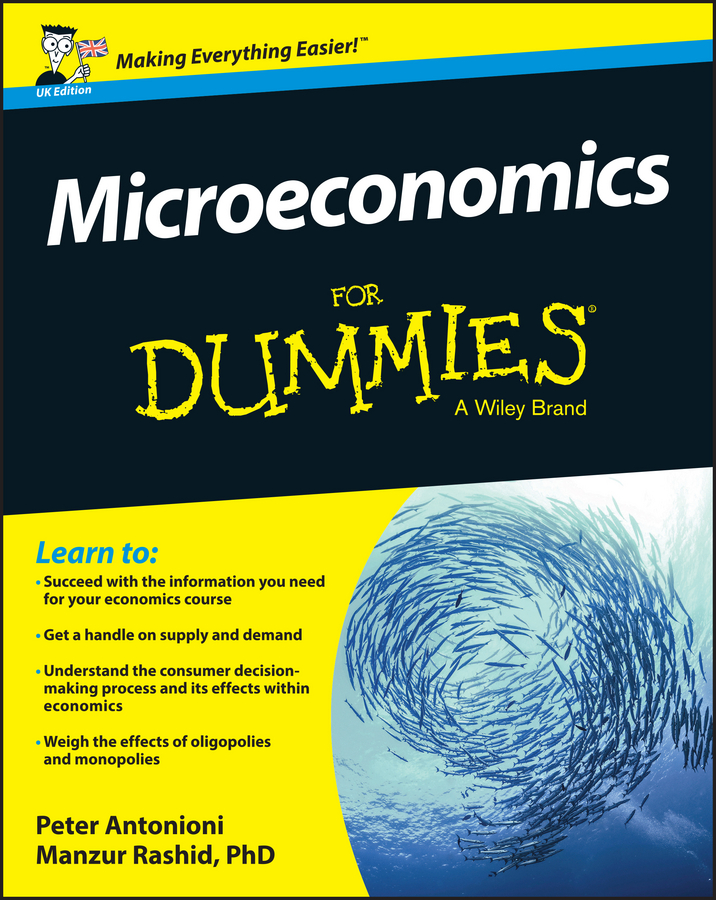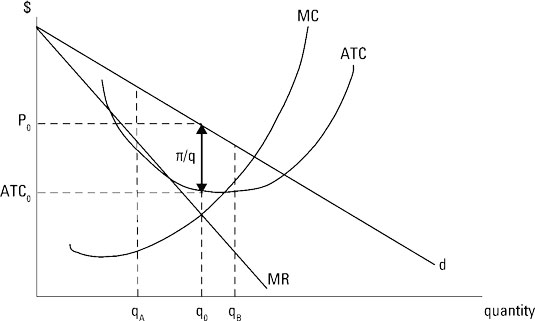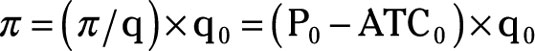##### Microeconomics For Dummies - UKManagerial economists have studied monopolistic competition to understand how to maximize profit in that economic model. Because a monopolistically competitive firm produces a differentiated good, short-run profit maximization requires the firm to determine both the profit-maximizing quantity and the good’s price. The illustration shows short-run profit maximization for a monopolistically competitive firm.

Differentiation leads to a downward-sloping demand curve for the monopolistically competitive firm. This curve is labeled d. Because the demand curve is downward sloping, the monopolistically competitive firm must lower price in order to sell more of the good. This lower price is charged for all units of the good sold.

Marginal revenue represents the change in total revenue that occurs when one additional unit of output is produced and sold. Because the firm must charge a lower price for every unit sold in order to sell one additional unit of the good, marginal revenue is lower than the price of the last unit sold. The marginal-revenue curve, thus, lies below the demand curve, as illustrated by the curve labeled MR.Marginal cost is the change in total cost that occurs when one additional unit of output is produced. Because of diminishing returns, marginal cost, MC, is upward-sloping. In addition, marginal cost passes through the minimum point of the average-total-cost curve, ATC.

The firm’s total profit increases if an additional unit of output adds more to revenue than it adds to cost. As long as the marginal revenue of an additional unit exceeds marginal cost, as is the case at qA, producing that unit increases the firm’s total profit, and the firm should continue to produce more.

On the other hand, if a unit of output adds more to cost than it adds to revenue, as with qB, or if marginal cost is greater than marginal revenue, producing that unit decreases the firm’s total profit. The firm needs to reduce production. The monopolistically competitive firm maximizes profit by producing the quantity of output associated with marginal revenue equals marginal cost.

The profit-maximizing quantity of output is represented by q0.

After determining the profit-maximizing quantity of output, the firm establishes the good’s price by going from that quantity, q0, to the demand curve and across to the vertical axis. The profit-maximizing price is P0.

The monopolistically competitive firm determines profit per unit by subtracting average total cost from price. In the illustration, profit per unit is represented by ð/q and equals price minus average total cost. Total profit, ð, equals profit per unit multiplied by the profit-maximizing quantity, or